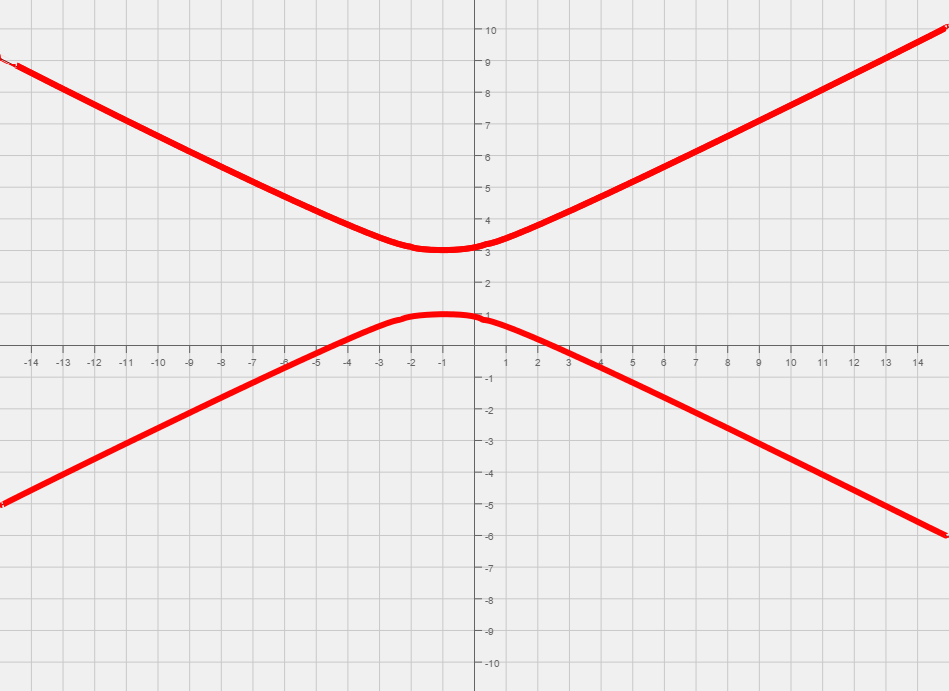# Difference between revisions of "005 Sample Final A, Question 16"

Question Graph the following,

$-x^{2}+4y^{2}-2x-16y+11=0$Foundations:
1) What type of function is this?
2) What can you say about the orientation of the graph?
1) Since both x and y are squared it must be a hyperbola or an ellipse. We can conclude that the graph is a hyperbola since $x^{2}$and   $y^{2}$have the different signs, one negative and one positive.
2) Since the $y^{2}$is positive, the hyperbola opens up and down.

Solution:

Step 1:
We start by completing the square twice, once for x and once for y. After completing the squares we end up with $-(x+1)^{2}+4(y-2)^{2}=4$Common Mistake: When completing the square we will end up adding numbers inside of parenthesis. So make sure you add the correct value to this other side. In this case we add -1, and 16 for completing the square with respect to x and y, respectively.
Before proceeding to the next step divide both sides by 4 to yield ${\frac {(y-2)^{2}}{1}}-{\frac {(x+1)^{2}}{4}}=1$Step 2:
Now that we have the equation that looks like a hyperbola, we can read off the center, (-1, 2). Now we will draw the box by moving up and down from the center by 1, and draw the horizontal lines y = 3 and y = 1. Do the same for the vertical lines by moving left and right from the center by 2, and draw the vertical lines x = -3 and x = 1. Now we have a box. Draw the two dashed lines that form diagonals of the box. These are the asymptotes. Since the hyperbola opens up and down we draw the corresponding parabolas, making sure to not cross the asymptotes.
The four vertices are: $(-3,-1),(3,-1),(0,1){\text{ and }}(0,-3)$.